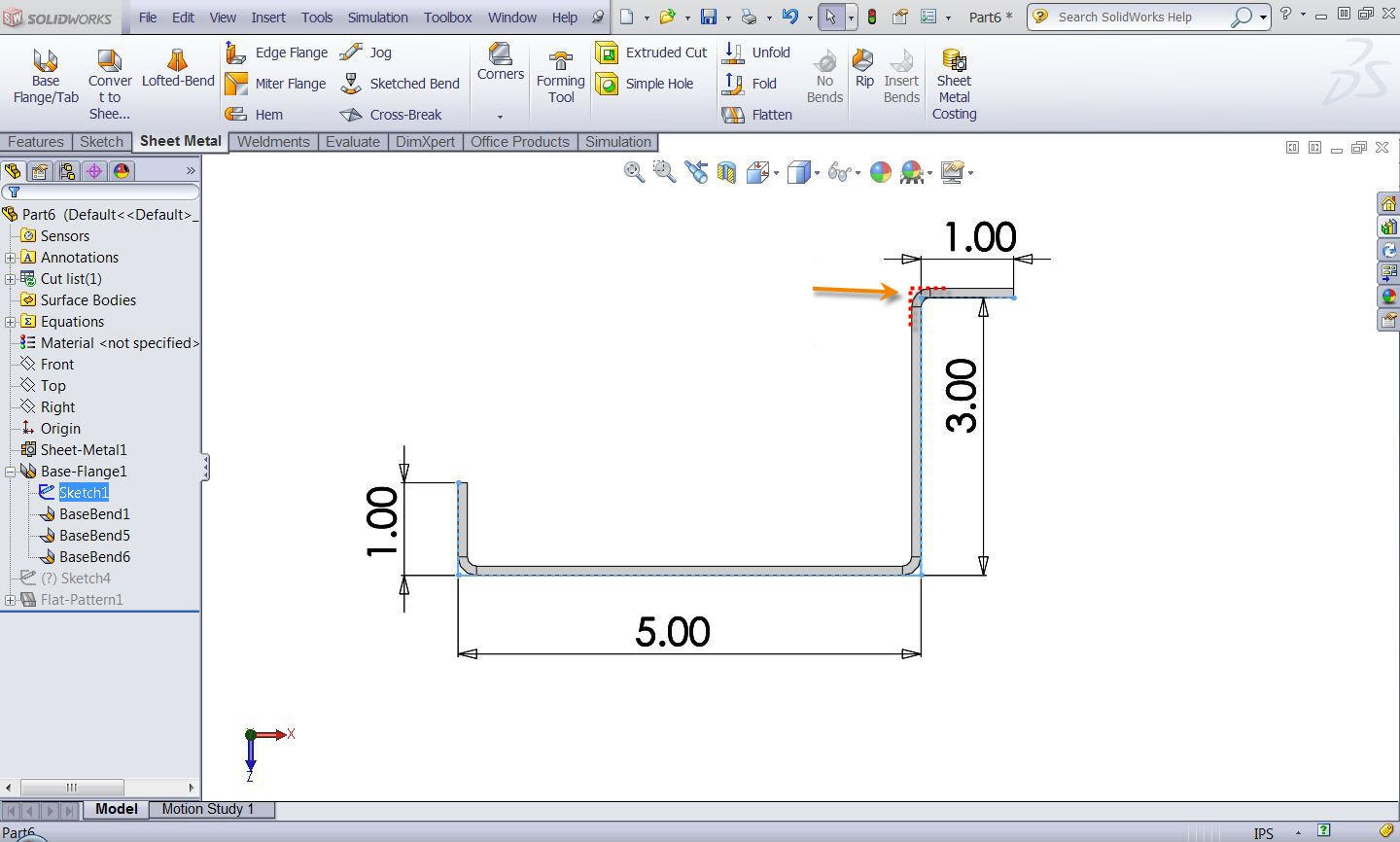# Sheet Metal Bend Deduction Calculations with Switching DirectionsArticle by Scott Durksen, CSWE updated January 15, 2013

###### Article

When using Bend Deductions in sheet metal, be careful when you have corners that change directions.  As shown at the bottom of the blog post Sheet Metal Bend Allowance Calculations, the Bend Deduction calculation is straight forward by taking all outside dimensions then subtracting the bend deduction values for each bend.

## All Bends in the Same Direction

Here is a simple example as a base flange with material inside the sketch.  The material thickness is 0.1″.  For simplicity, the Bend Deduction value has been set as 0.  Therefore the calculation of the flat pattern length is:

(Total Outside Dimensions) – (Bend Deduction * Number Of Bends)

= (1 + 5 + 3 + 1) – (0 * 3)

= 10″## Bends Switch Direction

But what happens if some of the bends are in the opposite direction?  Here is the same example with one of the sketch lines reversed.Notice how the reversed bend now has the material outside of the sketch corner.  The Bend Deduction calculation will be from the outside of the bend.  Therefore the Total Outside Dimensions will be larger than the sketch length.  For each corner that has material outside the sketch, it will also include twice the material thickness to calculate from the virtual outside corner.

(Total Outside Dimensions) – (Bend Deduction * Number Of Bends)

= (1 + 5 + 3.1 + 1.1) – (0 * 3)

= 10.2″

Make sure you are aware of this scenario when you start sketching multiple corners with Bend Deductions.

#### Get Certified SOLIDWORKS Services from Javelin